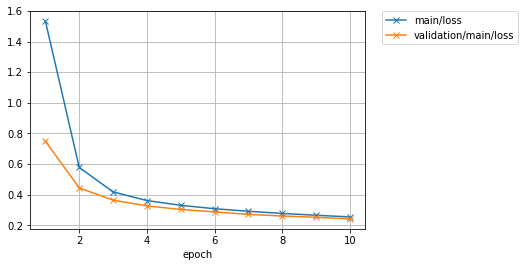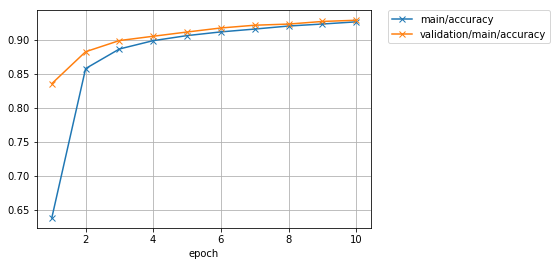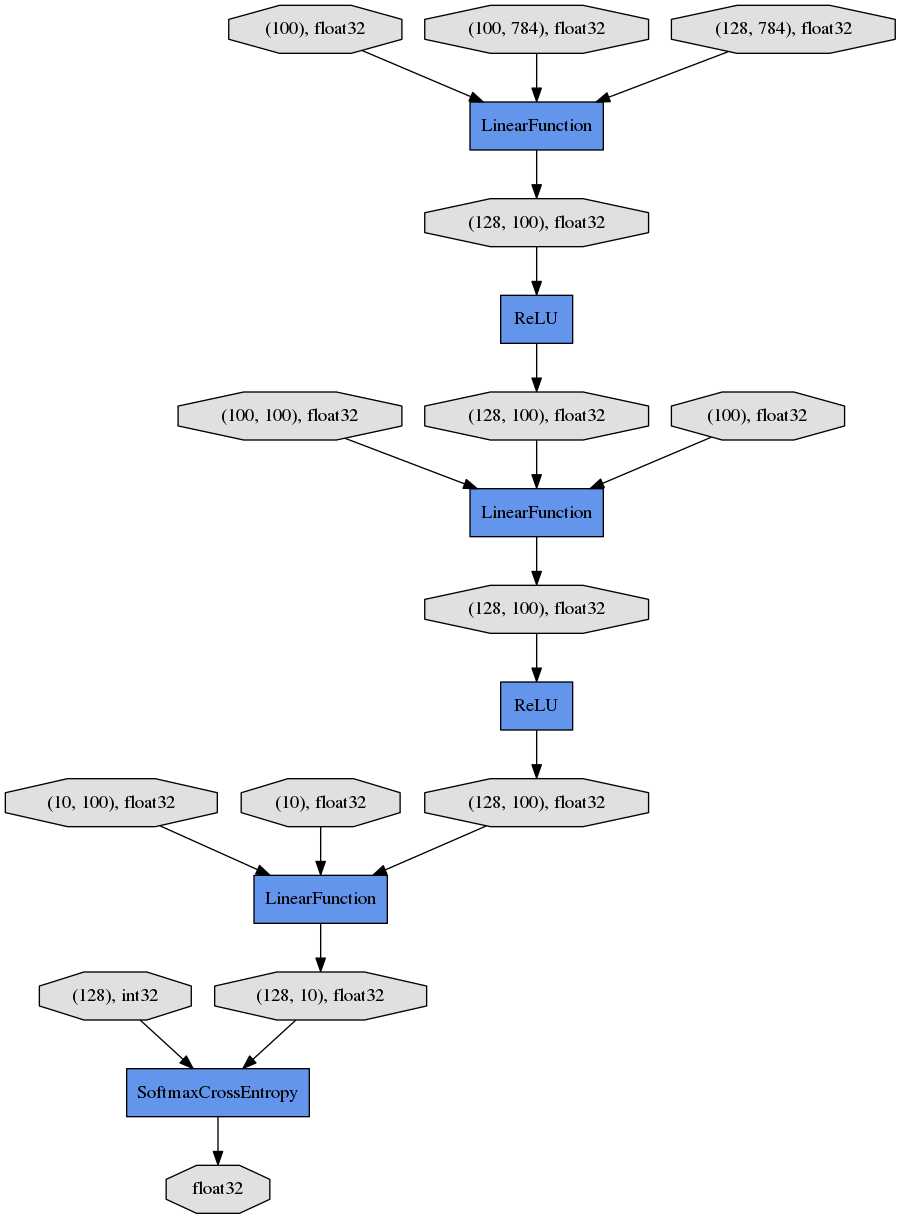# Let’s try using the Trainer feature¶

By using Trainer, you don’t need to write the training loop explicitly any more. Furthermore, Chainer provides many useful extensions that can be used with Trainer to visualize your results, evaluate your model, store and manage log files more easily.

This example will show how to use the Trainer to train a fully-connected feed-forward neural network on the MNIST dataset.

Note

If you would like to know how to write a training loop without using the Trainer, please check How to write a training loop in Chainer instead of this tutorial.

## 1. Prepare the dataset¶

Load the MNIST dataset, which contains a training set of images and class labels as well as a corresponding test set.

from chainer.datasets import mnist

train, test = mnist.get_mnist()


Note

You can use a Python list as a dataset. That’s because Iterator can take any object as a dataset whose elements can be accessed via [] accessor and whose lengh can be obtained with len() function. For example,

train = [(x1, t1), (x2, t2), ...]


a list of tuples like this can be used as a dataset.

There are many utility dataset classes defined in datasets. It’s recommended to utilize them in the actual applications.

For example, if your dataset consists of a number of image files, it would take a large amount of memory to load those data into a list like above. In that case, you can use ImageDataset, which just keeps the paths to image files. The actual image data will be loaded from the disk when the corresponding element is requested via [] accessor. Until then, no images are loaded to the memory to reduce memory use.

## 2. Prepare the dataset iterations¶

Iterator creates a mini-batch from the given dataset.

batchsize = 128

train_iter = iterators.SerialIterator(train, batchsize)
test_iter = iterators.SerialIterator(test, batchsize, False, False)


## 3. Prepare the model¶

Here, we are going to use the same model as the one defined in How to write a training loop in Chainer.

class MLP(Chain):

def __init__(self, n_mid_units=100, n_out=10):
super(MLP, self).__init__()
with self.init_scope():
self.l1 = L.Linear(None, n_mid_units)
self.l2 = L.Linear(None, n_mid_units)
self.l3 = L.Linear(None, n_out)

def __call__(self, x):
h1 = F.relu(self.l1(x))
h2 = F.relu(self.l2(h1))
return self.l3(h2)

gpu_id = 0  # Set to -1 if you use CPU

model = MLP()
if gpu_id >= 0:
model.to_gpu(gpu_id)


## 4. Prepare the Updater¶

Trainer is a class that holds all of the necessary components needed for training. The main components are shown below.Basically, all you need to pass to Trainer is an Updater. However, Updater contains an Iterator and Optimizer. Since Iterator can access the dataset and Optimizer has references to the model, Updater can access to the model to update its parameters.

So, Updater can perform the training procedure as shown below:

1. Retrieve the data from dataset and construct a mini-batch (Iterator)
2. Pass the mini-batch to the model and calculate the loss
3. Update the parameters of the model (Optimizer)

Now let’s create the Updater object !

max_epoch = 10

# Wrapp your model by Classifier and include the process of loss calculation within your model.
# Since we do not specify a loss funciton here, the default 'softmax_cross_entropy' is used.
model = L.Classifier(model)

# selection of your optimizing method
optimizer = optimizers.MomentumSGD()

# Give the optimizer a reference to the model
optimizer.setup(model)

# Get an updater that uses the Iterator and Optimizer
updater = training.StandardUpdater(train_iter, optimizer)


Note

Here, the model defined above is passed to Classifier and changed to a new Chain. Classifier, which in fact inherits from the Chain class, keeps the given Chain model in its predictor attribute. Once you give the input data and the corresponding class labels to the model by the () operator,

1. __call__() of the model is invoked. The data is then given to predictor to obtain the output y.
2. Next, together with the given labels, the output y is passed to the loss function which is determined by lossfun argument in the constructor of Classifier.
3. The loss is returned as a Variable.

In Classifier, the lossfun is set to softmax_cross_entropy() as default.

StandardUpdater is the simplest class among several updaters. There are also the ParallelUpdater and the MultiprocessParallelUpdater to utilize multiple GPUs. The MultiprocessParallelUpdater uses the NVIDIA NCCL library, so you need to install NCCL and re-install CuPy before using it.

## 5. Setup Trainer¶

Lastly, we will setup Trainer. The only requirement for creating a Trainer is to pass the Updater object that we previously created above. You can also pass a stop_trigger to the second trainer argument as a tuple like (length, unit) to tell the trainer when to stop the training. The length is given as an integer and the unit is given as a string which should be either epoch or iteration. Without setting stop_trigger, the training will never be stopped.

# Setup a Trainer
trainer = training.Trainer(updater, (max_epoch, 'epoch'), out='mnist_result')


The out argument specifies an output directory used to save the log files, the image files of plots to show the time progress of loss, accuracy, etc. when you use PlotReport extension. Next, we will explain how to display or save those information by using trainer Extension.

## 6. Add Extensions to the Trainer object¶

The Trainer extensions provide the following capabilites:

To use these wide variety of tools for your training task, pass Extension objects to the extend() method of your Trainer object.

trainer.extend(extensions.LogReport())
trainer.extend(extensions.snapshot(filename='snapshot_epoch-{.updater.epoch}'))
trainer.extend(extensions.snapshot_object(model.predictor, filename='model_epoch-{.updater.epoch}'))
trainer.extend(extensions.Evaluator(test_iter, model, device=gpu_id))
trainer.extend(extensions.PrintReport(['epoch', 'main/loss', 'main/accuracy', 'validation/main/loss', 'validation/main/accuracy', 'elapsed_time']))
trainer.extend(extensions.PlotReport(['main/loss', 'validation/main/loss'], x_key='epoch', file_name='loss.png'))
trainer.extend(extensions.PlotReport(['main/accuracy', 'validation/main/accuracy'], x_key='epoch', file_name='accuracy.png'))
trainer.extend(extensions.dump_graph('main/loss'))


### LogReport¶

Collect loss and accuracy automatically every epoch or iteration and store the information under the log file in the directory specified by the out argument when you create a Trainer object.

### snapshot()¶

The snapshot() method saves the Trainer object at the designated timing (defaut: every epoch) in the directory specified by out. The Trainer object, as mentioned before, has an Updater which contains an Optimizer and a model inside. Therefore, as long as you have the snapshot file, you can use it to come back to the training or make inferences using the previously trained model later.

### snapshot_object()¶

However, when you keep the whole Trainer object, in some cases, it is very tedious to retrieve only the inside of the model. By using snapshot_object(), you can save the particular object (in this case, the model wrapped by Classifier) as a separeted snapshot. Classifier is a Chain object which keeps the model that is also a Chain object as its predictor property, and all the parameters are under the predictor, so taking the snapshot of predictor is enough to keep all the trained parameters.

### dump_graph()¶

This method saves the structure of the computational graph of the model. The graph is saved in the Graphviz <http://www.graphviz.org/>_s dot format. The output location (directory) to save the graph is set by the :attr:~chainer.training.Trainer.out argument of Trainer.

### Evaluator¶

The Iterator that uses the evaluation dataset and the model object are required to use Evaluator. It evaluates the model using the given dataset (typically it’s a validation dataset) at the specified timing interval.

### PrintReport¶

It outputs the spcified values to the standard output.

### PlotReport¶

PlotReport plots the values specified by its arguments saves it as a image file which has the same naem as the file_name argument.

Each Extension class has different options and some extensions are not mentioned here. And one of other important feature is, for instance, by using the trigger option, you can set individual timings to fire the Extension. To know more details of all extensions, please take a look at the official document: Trainer extensions <reference/extensions.html>_.

## 7. Start Training¶

Just call run() method from Trainer object to start training.

trainer.run()

epoch       main/loss   main/accuracy  validation/main/loss  validation/main/accuracy  elapsed_time
1           1.53241     0.638409       0.74935               0.835839                  4.93409
2           0.578334    0.858059       0.444722              0.882812                  7.72883
3           0.418569    0.886844       0.364943              0.899229                  10.4229
4           0.362342    0.899089       0.327569              0.905558                  13.148
5           0.331067    0.906517       0.304399              0.911788                  15.846
6           0.309019    0.911964       0.288295              0.917722                  18.5395
7           0.292312    0.916128       0.272073              0.921776                  21.2173
8           0.278291    0.92059        0.261351              0.923457                  23.9211
9           0.266266    0.923541       0.253195              0.927314                  26.6612
10          0.255489    0.926739       0.242415              0.929094                  29.466


Let’s see the plot of loss progress saved in the mnist_result directory.Furthermore, let’s visualize the computaional graph saved with dump_graph() using Graphviz.

% dot -Tpng mnist_result/cg.dot -o mnist_result/cg.pngFrom the top to the bottom, you can see the data flow in the computational graph. It basically shows how data and parameters are passed to the Functions.

## 8. Evaluate a pre-trained model¶

Evaluation using the snapshot of a model is as easy as what explained in the How to write a training loop in Chainer.

import matplotlib.pyplot as plt

model = MLP()

# Show the output
x, t = test
plt.imshow(x.reshape(28, 28), cmap='gray')
plt.show()
print('label:', t)

y = model(x)

print('predicted_label:', y.argmax(axis=1))label: 7
predicted_label: 7


The prediction looks correct. Success!